# Erickson, Martin

## Transversal achievement game on a square grid ★★

Author(s): Erickson

Problem   Two players alternately write O's (first player) and X's (second player) in the unoccupied cells of an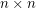grid. The first player (if any) to occupy a set ofcells having no two cells in the same row or column is the winner. What is the outcome of the game given optimal play?

Keywords: game

## Exact colorings of graphs ★★

Author(s): Erickson

Conjecture   For, let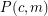be the statement that given any exact-coloring of the edges of a complete countably infinite graph (that is, a coloring withcolors all of which must be used at least once), there exists an exactly-colored countably infinite complete subgraph. Thenis true if and only if,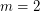, or.

Keywords: graph coloring; ramsey theory

## Square achievement game on an n x n grid ★★

Author(s): Erickson

Problem   Two players alternately write O's (first player) and X's (second player) in the unoccupied cells of angrid. The first player (if any) to occupy four cells at the vertices of a square with horizontal and vertical sides is the winner. What is the outcome of the game given optimal play? Note: Roland Bacher and Shalom Eliahou proved that every 15 x 15 binary matrix contains four equal entries (all 0's or all 1's) at the vertices of a square with horizontal and vertical sides. So the game must result in a winner (the first player) when n=15.

Keywords: game

## Sequence defined on multisets ★★

Author(s): Erickson

Conjecture   Define aarray of positive integers where the first row consists of some distinct positive integers arranged in increasing order, and the second row consists of any positive integers in any order. Create a new array where the first row consists of all the integers that occur in the first array, arranged in increasing order, and the second row consists of their multiplicities. Repeat the process. For example, starting with the array, the sequence is:->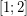->->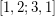->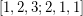->->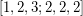->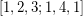->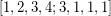->->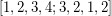->, and we now have a fixed point (loop of one array).

The process always results in a loop of 1, 2, or 3 arrays.

Keywords: multiset; sequence